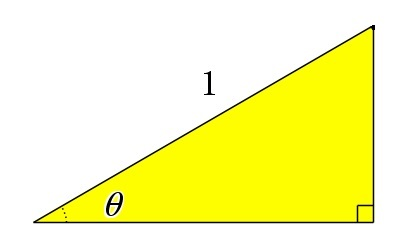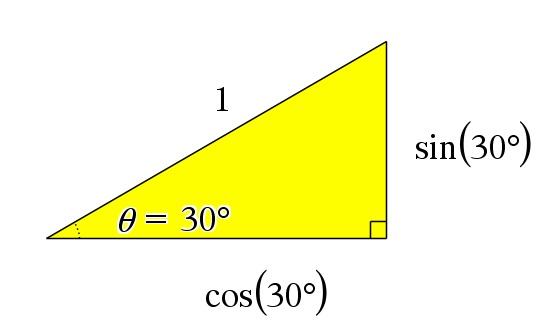# Trigonometry – an introduction

Up a level : IB Mathematics
Previous page : Derivatives
Next page : Functions, Quiz 1Trigonometry means measurement of something with three corners, i.e. on triangles.  We will here start with right angled triangles in particular.

Say you have a right angled triangle with the hypotenuse (the longest side) having the side 1, and one of the angles is say 30. Then let us draw another right angled triangle with the same angle and with the hypotenuse of length one. What can we now say about the two triangles? Hopefully you can see that they are congruent. That means that the right angled triangle with a hypotheses of length one is totally dependent on the given angle. And in particular, that its base and height (if placed as in the figure) is determined by the angle.If we change the angle, then these lengths will change too.  Let us label the angle θ (the Greek letter theta), then the height that we will call the opposite side since it is opposite to the angle θ is totally dependent on the angle θ. We say that it is a function of the angle.

As with say square root of x (that is a function of x) we want to give it a name. For say the square root of 16 we don’t want to have to say “the positive number such that the number multiplied by itself is 16”. We just say “the square root of 16”. In a similar way we don’t want to say “the length of the opposite side of a right angled triangle with the hypotenuse has the length one and the angle is 30˚  is….” We want to have a short form for this.

We say that the opposite side = sin(30˚).

The word sin (that is not pronounce like the word “sin” but rather lake “sin” in “sinuses”) is an abbreviation of the Latin word sinus meaning curve or bow. The word sinus has then turned to the word sine that is abbreviated as sin (wow, one letter less).

Observe that, by this definition, sin(θ) simply equals the length of the opposite side of a right angled triangle (where the hypotenuse has the length one) given the angle θ.

The base, that we will call the adjacent side, because it is adjacent to (next to) the angle, will also be a function of the angle. We say that the adjacent side =adj= cos(θ).   Here “cos” is an abbreviation of the word “cosine” where the “co” is the same as in words like “cooperate”.If we where to draw a triangle as the one above, with a hypotenuse of length 100 cm and we then would measure the length of the base (adjacent side) and the height (opposite site) we would find about 87 cm and 50 cm, and indeed if you take a calculator and calculate cos vs sine of 30˚, and indeed you will find about 0.87 and 0.5 (exactly). If you got something else your calculator angular setting is probably wrong. On a casio fx-9750 you should be in RUN-mode, then you press SHIFT, SET UP (the MENU key), then you go down to the angle setting and change it to DEG (degrees).

You can have a look at this Geogebra file.Up a level : IB Mathematics
Previous page : Derivatives
Next page : Functions, Quiz 1Last modified: Mar 31, 2019 @ 20:15